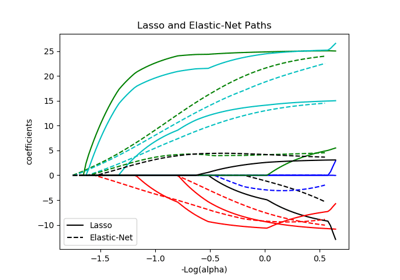/scikit-learn

# sklearn.linear_model.enet_path

sklearn.linear_model.enet_path(X, y, l1_ratio=0.5, eps=0.001, n_alphas=100, alphas=None, precompute=’auto’, Xy=None, copy_X=True, coef_init=None, verbose=False, return_n_iter=False, positive=False, check_input=True, **params) [source]

Compute elastic net path with coordinate descent

The elastic net optimization function varies for mono and multi-outputs.

For mono-output tasks it is:

1 / (2 * n_samples) * ||y - Xw||^2_2
+ alpha * l1_ratio * ||w||_1
+ 0.5 * alpha * (1 - l1_ratio) * ||w||^2_2


For multi-output tasks it is:

(1 / (2 * n_samples)) * ||Y - XW||^Fro_2
+ alpha * l1_ratio * ||W||_21
+ 0.5 * alpha * (1 - l1_ratio) * ||W||_Fro^2


Where:

||W||_21 = \sum_i \sqrt{\sum_j w_{ij}^2}


i.e. the sum of norm of each row.

Read more in the User Guide.

Parameters: X : {array-like}, shape (n_samples, n_features) Training data. Pass directly as Fortran-contiguous data to avoid unnecessary memory duplication. If y is mono-output then X can be sparse. y : ndarray, shape (n_samples,) or (n_samples, n_outputs) Target values l1_ratio : float, optional float between 0 and 1 passed to elastic net (scaling between l1 and l2 penalties). l1_ratio=1 corresponds to the Lasso eps : float Length of the path. eps=1e-3 means that alpha_min / alpha_max = 1e-3 n_alphas : int, optional Number of alphas along the regularization path alphas : ndarray, optional List of alphas where to compute the models. If None alphas are set automatically precompute : True | False | ‘auto’ | array-like Whether to use a precomputed Gram matrix to speed up calculations. If set to 'auto' let us decide. The Gram matrix can also be passed as argument. Xy : array-like, optional Xy = np.dot(X.T, y) that can be precomputed. It is useful only when the Gram matrix is precomputed. copy_X : boolean, optional, default True If True, X will be copied; else, it may be overwritten. coef_init : array, shape (n_features, ) | None The initial values of the coefficients. verbose : bool or integer Amount of verbosity. return_n_iter : bool whether to return the number of iterations or not. positive : bool, default False If set to True, forces coefficients to be positive. (Only allowed when y.ndim == 1). check_input : bool, default True Skip input validation checks, including the Gram matrix when provided assuming there are handled by the caller when check_input=False. **params : kwargs keyword arguments passed to the coordinate descent solver. alphas : array, shape (n_alphas,) The alphas along the path where models are computed. coefs : array, shape (n_features, n_alphas) or (n_outputs, n_features, n_alphas) Coefficients along the path. dual_gaps : array, shape (n_alphas,) The dual gaps at the end of the optimization for each alpha. n_iters : array-like, shape (n_alphas,) The number of iterations taken by the coordinate descent optimizer to reach the specified tolerance for each alpha. (Is returned when return_n_iter is set to True).

#### Notes

For an example, see examples/linear_model/plot_lasso_coordinate_descent_path.py.

## Examples using sklearn.linear_model.enet_pathLasso and Elastic Net

© 2007–2018 The scikit-learn developers